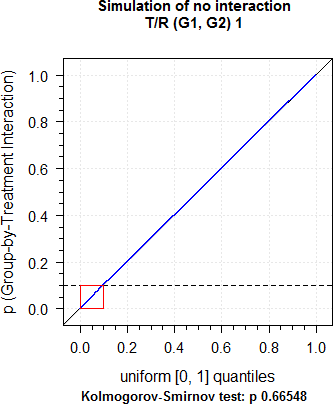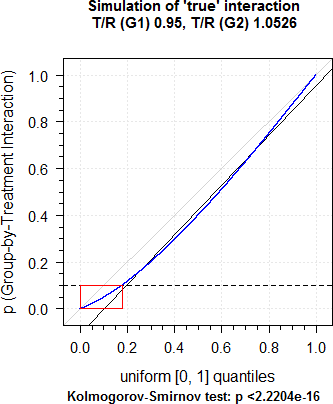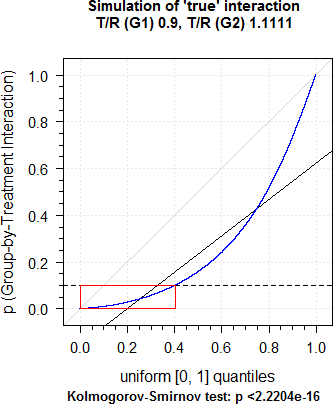## Simulations [Regulatives / Guidelines]

Hi mittyri,

❝ ❝ The idea behind the Group-by-Treatment interaction is that the T/R in one group is different from the other (i.e., we have collinearity with a “hidden” variable). Therefore, simulate a group of subjects with T/R 0.95 and another one with T/R 0.95–1 (CV ad libitum). Merge them to get a “study”. Run model 1 and check the p-value of the Group-by-Treatment interaction. With the simple model you should expect T/R 1.

❝ seems to be reasonable, but I do not see why the power is low?

Good question. Next question?

I performed simulations (100,000 2×2×2 studies each for conditions a. and b. specified below). Two groups of 16 subjects each, CV 30%, no period and sequence effects. 32 subjects should give power 81.52% for T/R 1. If the Group-by-Treatment interaction is not significant (p ≥0.1) in model 1, the respective study is evaluated by model 2 (pooled data) or both groups by model 3 otherwise. All studies are evaluated by model 3 (pooled data). The listed PE is the geometric mean of passing studies’ PEs.
1. T/R in group 1 0.95, T/R in group 2 0.95–1
(i.e., ‘true’ Group-by-Treatment interaction):
Model 1: p(G×T) <0.1 in 17.91% of studies. Evaluation of studies with p(G×T) <0.1 (Groups):   passed model 3 (1)      :  1.42% (of tested); PE  98.69%                                   range of PEs: 92.45% to 107.63%   passed model 3 (2)      :  1.64% (of tested); PE 100.99%                                   range of PEs: 93.99% to 108.23%   passed model 3 (1 and 2):  0.00% (of tested) Evaluation of studies with p(G×T) ≥0.1 (pooled):   passed model 2          : 66.47% (overall)                             80.97% (of tested); PE  99.97%                                   range of PEs: 86.36% to 114.27% Studies passing any of model 2 or 3: 67.02% Criteria for simple model fulfilled:   passed model 3          : 80.95%;             PE  99.98%                                   range of PEs: 86.36% to 114.68%

2. T/R in both groups 1.00
(i.e., no Group-by-Treatment interaction):
Model 1: p(G×T) <0.1 in 9.79% of studies. Evaluation of studies with p(G×T) <0.1 (Groups):   passed model 3 (1)      :  1.86% (of tested); PE 100.28%                                   range of PEs: 93.09% to 108.40%   passed model 3 (2)      :  1.87% (of tested); PE 100.01%                                   range of PEs: 92.18% to 108.41%   passed model 3 (1 and 2):  0.00% (of tested) Evaluation of studies with p(G×T) ≥0.1 (pooled):   passed model 2          : 73.33% (overall)                             81.28% (of tested); PE  99.98%                                   range of PEs: 86.36% to 114.68% Studies passing any of model 2 or 3: 73.69% Criteria for simple model fulfilled:   passed model 3          : 81.40%;             PE  99.98%                                   range of PEs: 86.36% to 115.15%
IMHO, equal groups sizes are problematic. What if one group passes and the other fails? Even if one is fishy and present only the passing one, assessors likely would ask for the other one and make a conservative decision. Hoping that both groups will pass is simply futile.

Lessons learned:
If we test at the 10% level and there is no true Group-by-Treatment interaction we will find a significant effect at ~ the level of the test – as expected (b). Hurray, false positives!
On the other hand, if there is one, we will detect it (a).
The percentage of studies passing in models 2 and 3 are similar. Theoretically in model 2 it should be slightly lower than in model 3 (one degree of freedom of the treatment effect less). However, overall power is seriously compromised.

Slowly I get the impression that the evaluation of groups (by model 3) is not a good idea. If there is a true Group-by-Treatment interaction why the heck should the PE (say in the largest group) be unbiased? I would rather say that if one believes that a Group-by-Treatment interaction really exists (I don’t) and the test makes sense (I don’t) evaluation (of the largest group) by model 3 should not be performed. Consequently ~⅒ of (otherwise passing) studies would go into the waste bin. Didn’t I say that before?

The distribution of p-values should be uniform.
Looks good for b.Min.   1st Qu.    Median      Mean   3rd Qu.      Max. 0.0000011 0.2517777 0.5002957 0.5008763 0.7508297 0.9999974

Interesting shape for a.Min.   1st Qu.    Median      Mean   3rd Qu.      Max. 0.0000001 0.1562932 0.3991516 0.4306846 0.6868190 0.9999981

If you prefer more extreme stuff: T/R in group 1 0.90, T/R in group 2 0.90–1

Model 1: p(G×T) <0.1 in 40.35% of studies. Evaluation of studies with p(G×T) <0.1 (Groups):   passed model 3 (1)      :  1.09% (of tested); PE  98.76%                                   range of PEs: 91.69% to 105.97%   passed model 3 (2)      :  1.06% (of tested); PE 101.40%                                   range of PEs: 94.58% to 108.34%   passed model 3 (1 and 2):  0.00% (of tested) Evaluation of studies with p(G×T) ≥0.1 (pooled):   passed model 2          : 47.74% (overall)                             80.03% (of tested); PE  99.98%                                   range of PEs: 87.24% to 114.13% Studies passing any of model 2 or 3: 48.60% Criteria for simple model fulfilled:   passed model 3          : 79.45%;             PE  99.99%                                   range of PEs: 87.24% to 114.13%Min. 1st Qu.  Median    Mean 3rd Qu.    Max. 0.00000 0.03962 0.15648 0.26602 0.42742 0.99997

PS: The code seems to work – at least for the pooled model 3. Comparisons of powers
power.TOST(...)                0.815152 power.TOST.sim(..., nsims=1e5) 0.81437 power.TOST.sim(..., nsims=1e6) 0.815127 My code (nsims=1e5)            0.81402 My code (nsims=1e6)            0.81551

Dif-tor heh smusma 🖖🏼 Довге життя Україна!Helmut SchützThe quality of responses received is directly proportional to the quality of the question asked. 🚮
Science QuotesIng. Helmut Schütz